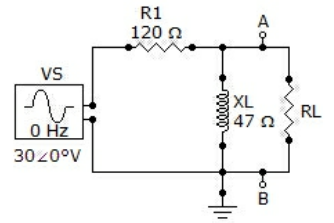Q 1. Determine VTH for the circuit external to RL in the given figure.(A) 135 ∠63.4° V
(B) 13.5 ∠63.4° V
(C) 13.5 ∠0° V
(D) 135 ∠0° V

Q 2. A Thevenin ac equivalent circuit always consists of an equivalent ac voltage source and an equivalent capacitance.

(A) True
(B) False

Q 3. Referring to the given circuit, how much power, in watts, is delivered to the speaker at the determined frequency if VS = 4.5 VRMS?(A) 226 mW
(B) 2.26 mW
(C) 4.24 mW
(D) 424 mW

Q 4. Like Thevenin’s theorem, Norton’s theorem provides a method of reducing a more complex circuit to a simpler, more manageable form for analysis.

(A) True
(B) False

Q 5. If two currents are in the same direction at any instant of time in a given branch of a circuit, the net current at that instant

(A) Is zero
(B) Is the sum of the two currents
(C) Is the difference between the two currents
(D) Cannot be determined

Q 6. Referring to the given circuit, what is ZTH if R1 is changed to 220 Ω?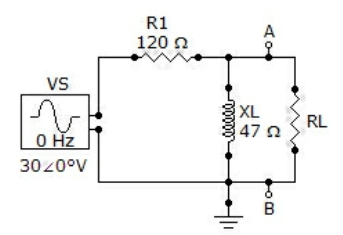(A) 225 ∠12.1° Ω
(B) 225 ∠77.9° Ω
(C) 46 ∠77.9° Ω
(D) 46 ∠12.1° Ω

Q 7. Norton’s theorem provides a method for the reduction of any ac circuit to an equivalent form consisting of an equivalent voltage source in series with an equivalent impedance.

(A) True
(B) False

Q 8. Thevenin’s theorem provides a method for the reduction of any ac circuit to an equivalent form consisting of an equivalent current source in parallel with an equivalent impedance.

(A) True
(B) False

Q 9. Norton’s theorem gives

(A) An equivalent current source in parallel with an equivalent impedance
(B) An equivalent current source in series with an equivalent impedance
(C) An equivalent voltage source in parallel with an equivalent impedance
(D) An equivalent voltage source in series with an equivalent impedance

Q 10. One circuit is equivalent to another, in the context of Thevenin’s theorem, when the circuits produce the same voltage.

(A) True
(B) False

Q 11. Referring to the given circuit, determine ZTH as seen by RL.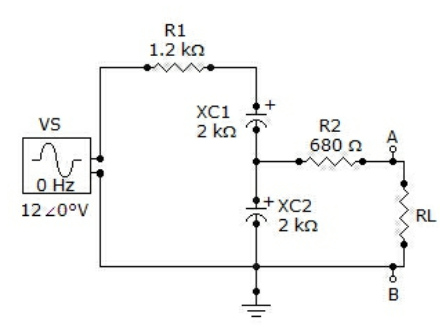(A) 1444 ∠-48.5° Ω
(B) 4176 ∠-73.3° Ω
(C) 956 ∠-48.5° Ω
(D) 1444 ∠-73.3° Ω

Q 12. Referring to the given circuit, what is VTH if VS = 12 ∠0° V?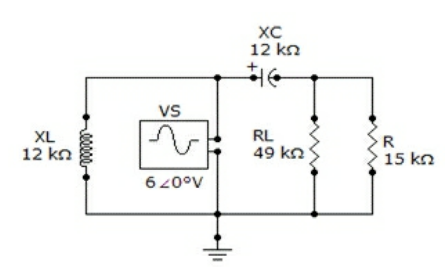(A) 4.69 ∠38.7° V
(B) 9.38 ∠38.7° V
(C) 12 ∠0° V
(D) 6 ∠0° V

Q 13. Determine the frequency at which the maximum power is transferred from the amplifier to the speaker in the given figure.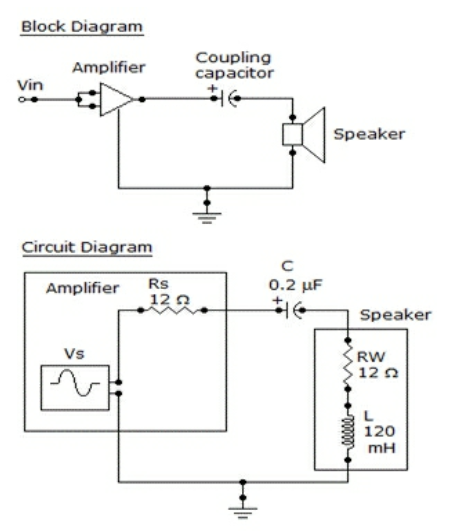(A) 1,027 Hz
(B) 10,270 Hz
(C) 6,330 Hz
(D) 63,330 Hz

Q 14. For the circuit given, determine the Thevenin voltage as seen by RL.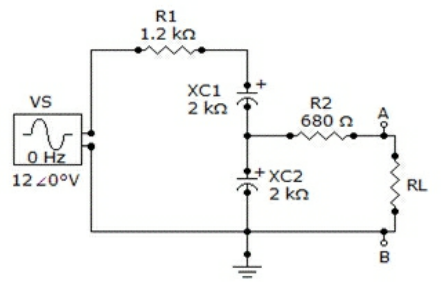(A) 0.574 ∠16.7° V
(B) 5.74 ∠16.7° V
(C) 0.574 ∠-16.7° V
(D) 5.74 ∠-16.7° V

Q 15. In order to get maximum power transfer from a capacitive source, the load must have an impedance that is the complex conjugate of the source impedance.

(A) True
(B) False

Q 16. The Norton equivalent current is

(A) The current through the load
(B) The open-current from the source
(C) The short circuit current
(D) None of the above

Q 17. Determine VTH when R1 is 180 Ω and XL is 90 Ω.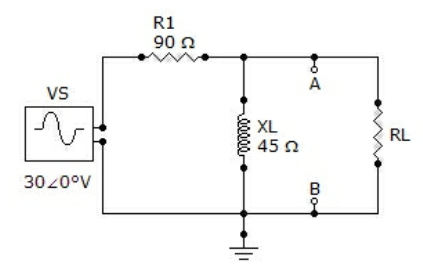(A) 135∠63.4° V
(B) 13.5∠63.4° V
(C) 12.2∠0° V
(D) 122∠0° V

Q 18. An equivalent circuit is one that produces the same voltage and current to a given load as the original circuit that it replaces.

(A) True
(B) False

Q 19. The Thevenin equivalent voltage is

(A) Equal to the source voltage
(B) The same as the load voltage
(C) The open circuit voltage
(D) None of the above

Q 20. Referring to the given circuit, find ZTH if R is 15 kΩ and RL is 38 kΩ.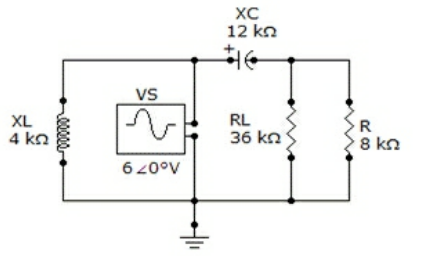(A) 89.82 ∠-51.3° kΩ
(B) 19.2 ∠-38.3° kΩ
(C) 9.38 ∠-51.3° kΩ
(D) 180 ∠-38.3° kΩ

Q 21. In an ac circuit, power to the load peaks at the frequency at which the load impedance is the complex conjugate of the output impedance.

(A) True
(B) False

Q 22. In order to get maximum power transfer from a capacitive source, the load must

(A) Have a capacitive reactance equal to circuit resistance
(B) Have an impedance that is the complex conjugate of the source impedance
(C) Be as capacitive as it is inductive
(D) None of the above

Q 23. Determine VTH if R1 is changed to 3.3 kΩ.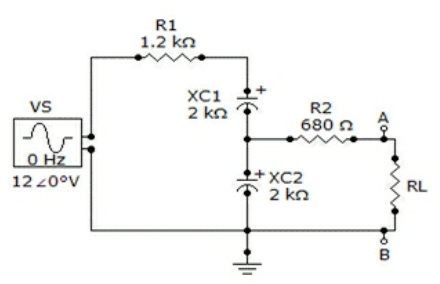(A) 0.574 ∠16.7° V
(B) 4.63 ∠16.7° V
(C) 4.63 ∠39.5° V
(D) 0.463 ∠39.5° V

Q 24. For the given circuit, find VTH for the circuit external to RL.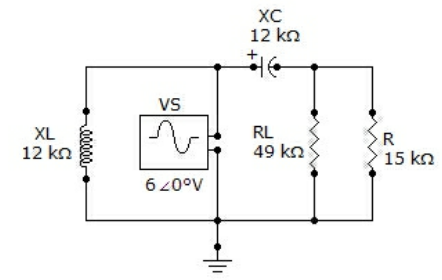(A) 4.69 ∠51.3° V
(B) 4.69 ∠38.7° V
(C) 469 ∠38.7° mV
(D) 6 ∠0° V

Q 25. The superposition theorem is useful for the analysis of single-source circuits.

(A) True
(B) False

Q 26. For the circuit shown, determine ZTH for the portion of the circuit external to RL.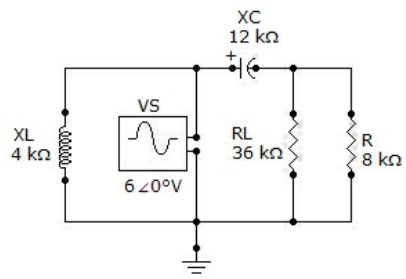(A) 66.7 ∠-33.7° kΩ
(B) 6.67 ∠-333.7° kΩ
(C) 14.4 ∠-56.3° kΩ
(D) 1.44 ∠-33.7° kΩ

Q 27. The two basic components of a Thevenin equivalent ac circuit are

(A) The equivalent voltage source and the equivalent series impedance
(B) The equivalent voltage source and the equivalent series resistance
(C) The equivalent voltage source and the equivalent parallel impedance
(D) The equivalent voltage source and the equivalent parallel resistance

Q 28. Referring to the given figure, determine ZTH as seen by RL if R1 is changed to 3.3 kΩ.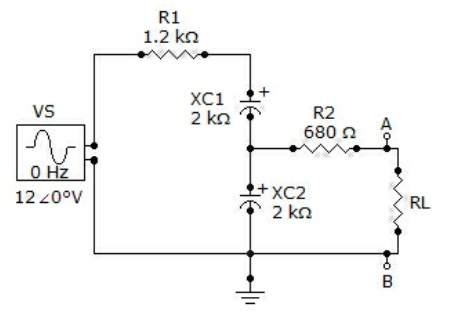(A) 1488 ∠-70.7° Ω
(B) 3859 ∠-31.2° Ω
(C) 5180 ∠-50.5° Ω
(D) 1828 ∠-50.2° Ω

Q 29. Referring to the given circuit, find ZTH if VS is 18∠0° V.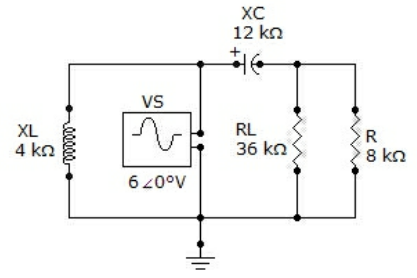(A) 9.82 ∠-51.3° kΩ
(B) 9.38 ∠-51.3° kΩ
(C) 180 ∠-38.3° kΩ
(D) 19.2 ∠-38.3° kΩ

Q 30. The superposition theorem is useful for circuit analysis only in ac circuits.

(A) True
(B) False

Q 31. Referring to the given circuit, L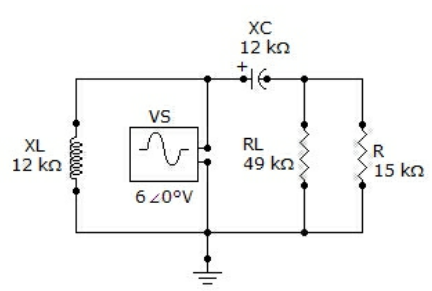(A) Must be in parallel with RL
(B) Must be placed in parallel with VS
(C) Must have a reactance equal to XC
(D) Has no effect on the result

Q 32. In applying the superposition theorem,

(A)  The sources are considered one at a time with all others replaced by their internal impedance
(B) All sources are considered independently
(C) All sources are considered simultaneously
(D)  The sources are considered one at a time with all others replaced by their internal resistance